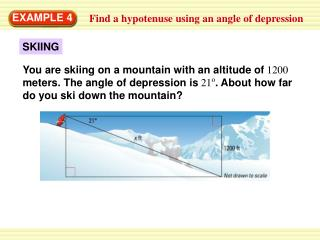DownloadDownload PresentationEXAMPLE 4

# EXAMPLE 4

Download Presentation## EXAMPLE 4

- - - - - - - - - - - - - - - - - - - - - - - - - - - E N D - - - - - - - - - - - - - - - - - - - - - - - - - - -
##### Presentation Transcript

1. You are skiing on a mountain with an altitude of 1200 meters. The angle of depression is 21o. About how far do you ski down the mountain? EXAMPLE 4 Find a hypotenuse using an angle of depression SKIING

2. = opp hyp 1200 = x xsin 21o 1200. x = sin21o 1200. x 0.3584 ANSWER x 3348.2 You ski about 3348 meters down the mountain. EXAMPLE 4 Find a hypotenuse using an angle of depression SOLUTION sin 21o Write ratio for sine of 21o. sin 21o Substitute. = 1200 Multiply each side by x. Divide each side by sin 21o Use a calculator to find sin21o Simplify.

3. = opp hyp 1200 = x xsin 28o 1200. x = sin28o for Example 4 GUIDED PRACTICE 6 WHAT IF ? Suppose the angle of depression in Example 4 is 28°. About how far would you ski? SOLUTION sin 28o Write ratio for sine of 28o. sin 28o Substitute. = 1200 Multiply each side by x. Divide each side by sin 28o

4. 1200. x 0.4694 x 2556m for Examples 4 GUIDED PRACTICE Use a calculator to find sin28o Simplify.